Ex 4.1

Chapter 4 Class 11 Mathematical Induction (Deleted)
Serial order wise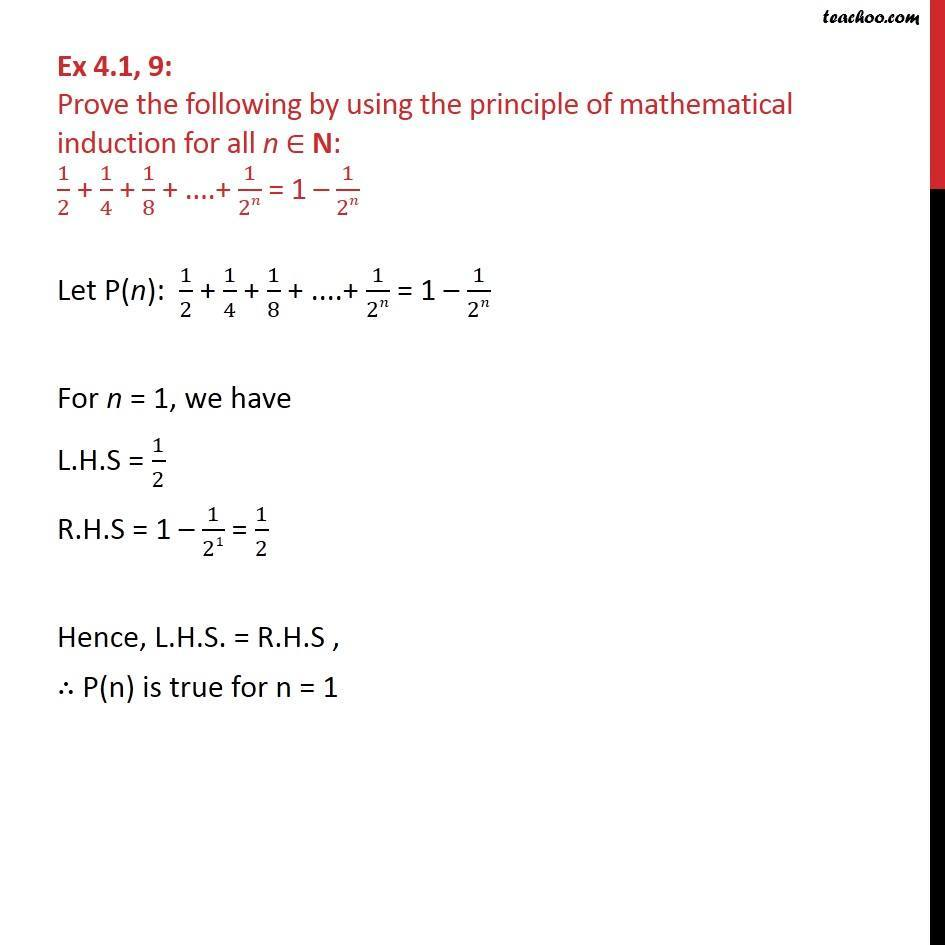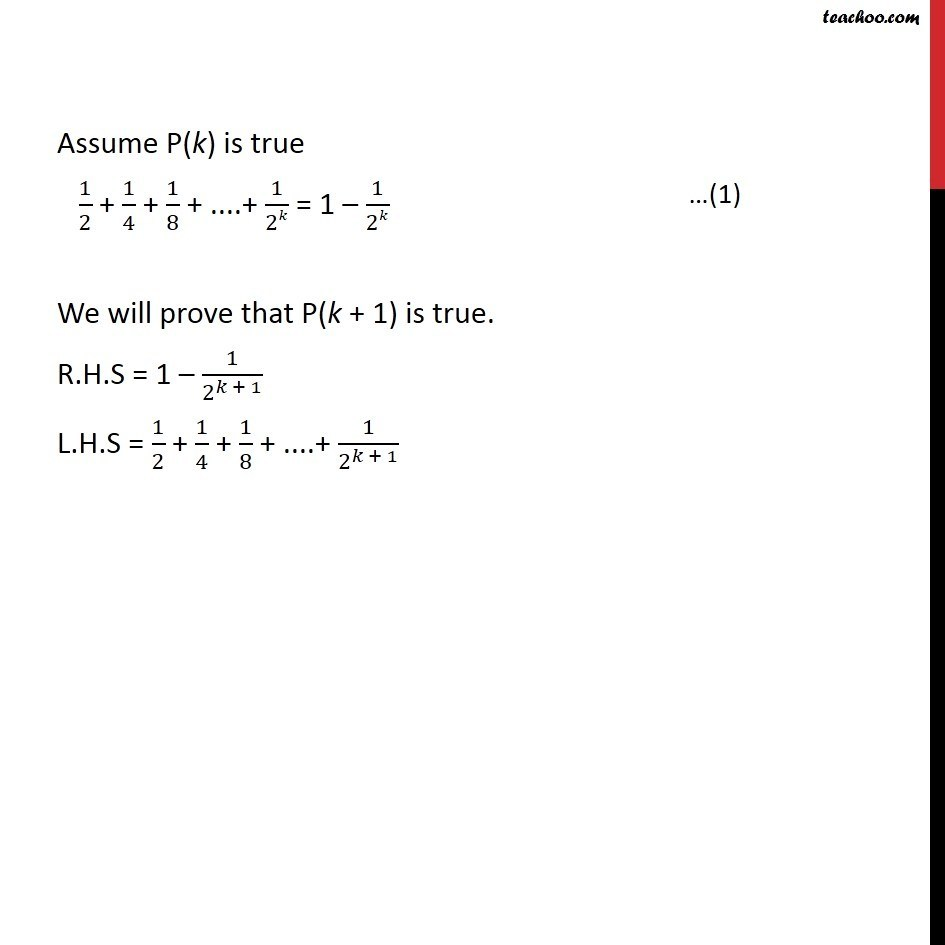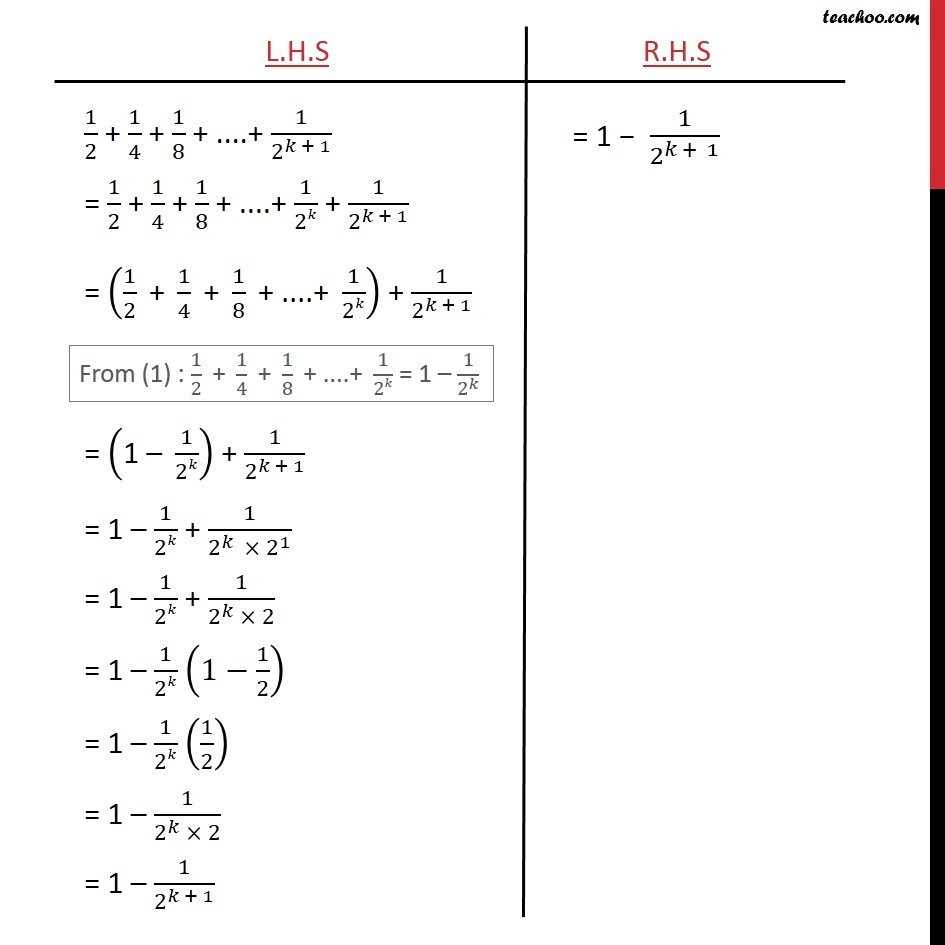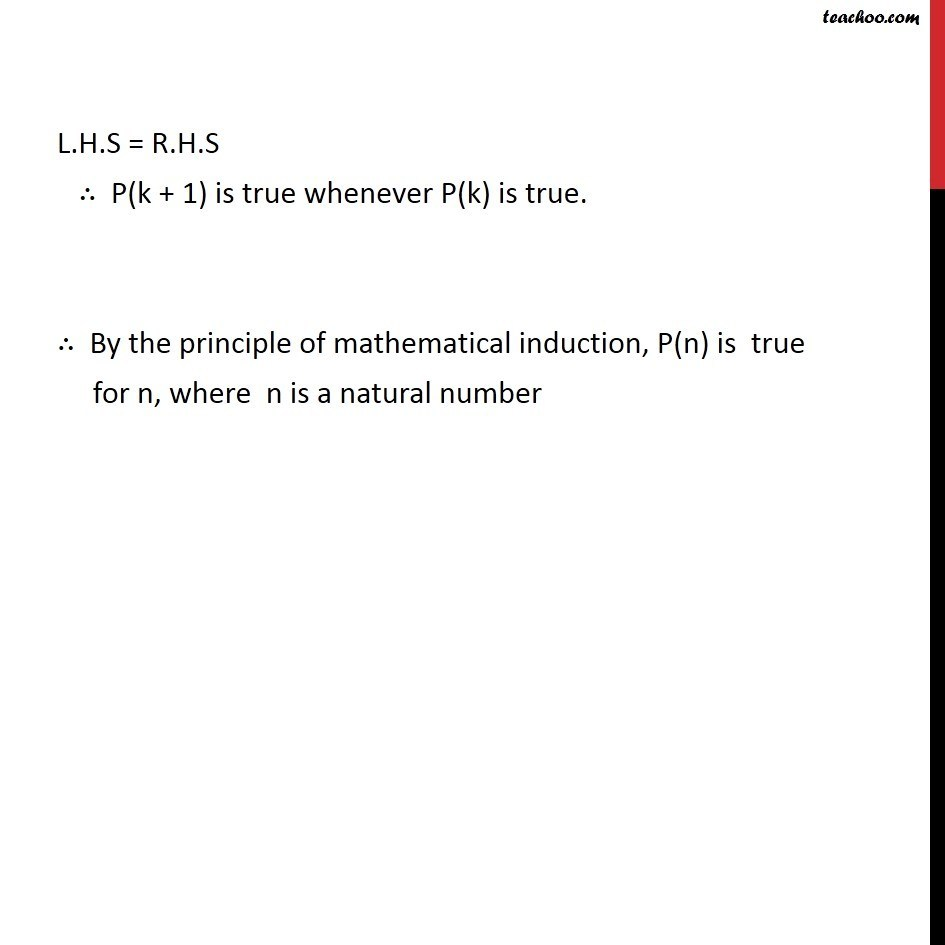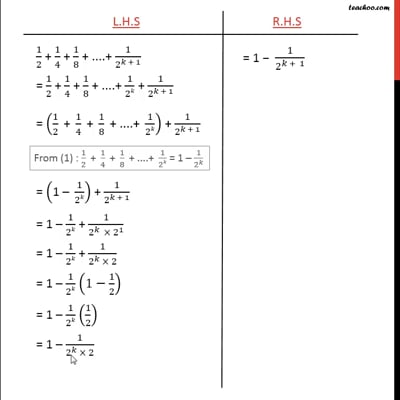This video is only available for Teachoo black users

### Transcript

Ex 4.1, 9: Prove the following by using the principle of mathematical induction for all n ∈ N: 1/2 + 1/4 + 1/8 + ....+ 1/2𝑛 = 1 – 1/2𝑛 Let P(n): 1/2 + 1/4 + 1/8 + ....+ 1/2𝑛 = 1 – 1/2𝑛 For n = 1, we have L.H.S = 1/2 R.H.S = 1 – 1/21 = 1/2 Hence, L.H.S. = R.H.S , ∴ P(n) is true for n = 1 Assume P(k) is true 1/2 + 1/4 + 1/8 + ....+ 1/2𝑘 = 1 – 1/2𝑘 We will prove that P(k + 1) is true. R.H.S = 1 – 1/2^(𝑘 + 1) L.H.S = 1/2 + 1/4 + 1/8 + ....+ 1/2^(𝑘 + 1) L.H.S = R.H.S ∴ P(k + 1) is true whenever P(k) is true. ∴ By the principle of mathematical induction, P(n) is true for n, where n is a natural number